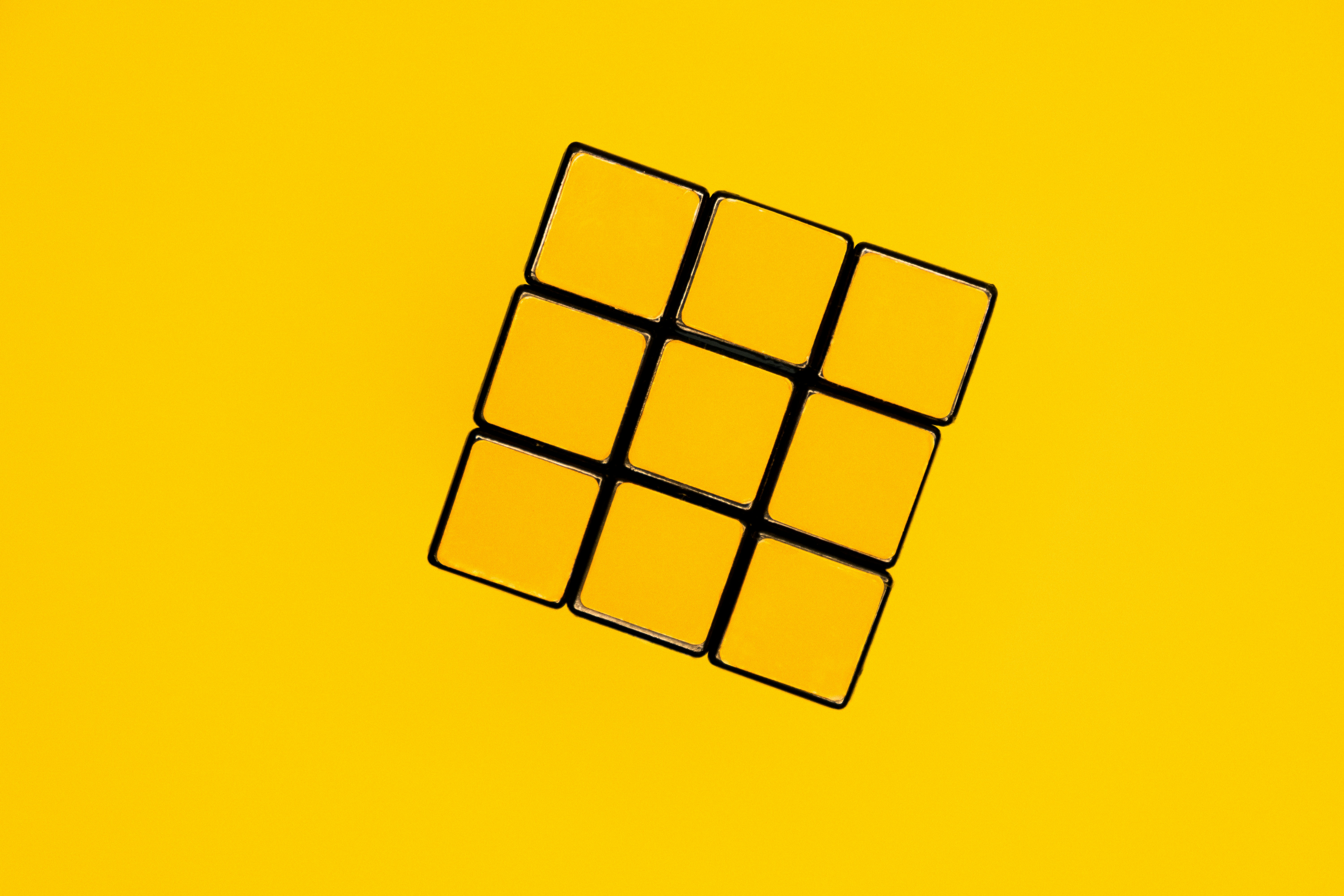Arsalan Wasim

# The Convolution OperatorLet's say that you want to detect features of a certain group of objects or class in other words. The features can be the edges, textures, patterns and objects in the frame or frames. A convolution operation can learn features in the form of feature maps. Let us explore more.

A convolution is an operation of applying a filter to an input. The input can be either two-dimensional or three-dimensional such as a two dimensional matrix of numbers (rows, columns) or an image with (rows, columns, channel). The result of the convolution operation is that the input is downsampled but the feature of interest is shown distinctly; let us see the way it works:

Let's suppose we have an input two-dimensional matrix:The filter is called a convolutional kernel and is a two-dimensional matrix, the kernel specifies the operation to be performed, in this example we will use a horizontal line detector kernel, which is represented as:This kernel, which has size (3 rows, 3 columns), is applied to the input which is of size ( 4 rows , 6 columns).

As it can be seen that the kernel is first applied to the top left corner of the input 2D matrix so the output is:

0x0 + 1x1 + 1x0 +0x0 + 1x1 + 1x0 + 0x0 + 1x1 + 0x0 = 0+1+0+1+0+0+1+0 =3

Now the kernel is moved to the right with a stride of one, stride defines the amount of pixels the filter has to move, in this case, it is one, so the output of convolution is:

1x0+ 1x1+ 0x0+ 1x0+ 1x1+ 0x0 + 0x1+ 1x1 + 0x0 = 0+1+0+0+1+0+0+1+0 = 3

The result of total operation is:As you can see the horizontal line filter has successfully calculated the horizontal line eliminating the zeros, larger input results in more convolution operations to be performed. In a convolutional neural network, there are many other filters like this to extract deep rich features.